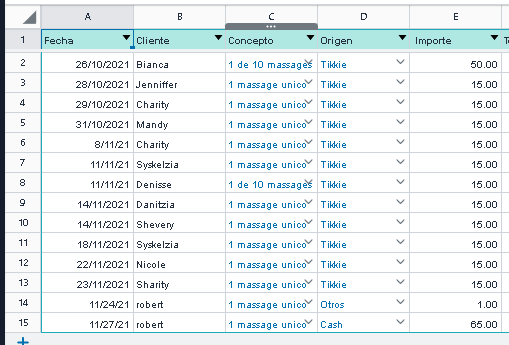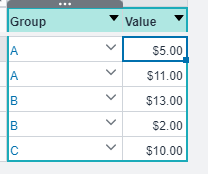# Help formula... noob here!

Hi there, i am trying to create a dashboard of data from my tables. but i cant find the right way to do it...

my table:My Problem :

So what is the right formula? in order to get my totals :

Idk how to explained... hope someone understand and help me, i want to learn.

Welcome to the Honeycode community, @RobertKirk!

I'm not entirely sure what you're looking for, but I'm guessing you want to sum values in a table, grouped by a specific column.

Below is a demo I've written up. Consider the following table:To sum all the values in the column 'Value' where 'Group' is equal to some value, use the following formula:

``````=SUMIF(Table1[Group],"A",Table1[Value])
``````

If you want to simply sum all of the 'Value' entries in the table, you can use the following formula:

``````=SUM(Table1[Value])
``````

This should give you something like this for the above demo table:

That is, in your case, the code would look something along the lines of:

``````=SUMIF(TableName[Origen], "Tikkie", TableName[Importe])
``````

Or, if you want your code to be more robust (seeing as how you are using rowlinks, something like below should work (this one hasn't been tested):

``````=SUMIF(TableName[Origen], FINDROW(OrigenTable, "OrigenTable[Origen] = %", "Tikkie"), TableName[Importe])
``````
2 Likes

This topic was automatically closed 3 days after the last reply. New replies are no longer allowed.